×

Search anything:

# K-th permutation of first N integers

#### Algorithms List of Mathematical AlgorithmsIn this article, we will understand and explore about the concept of generating k-th lexicographical permutation of first N integers. There are O(N!) permutations but you can find it in O(N) time.

• Permutation sequence
• kth permutation of first n integers
• Complexity analysis

This is similar to Leetcode problem 60. Permutation Sequence. Let us get started with this problem.

# Permutation Sequence

Literally, permutation is the arrangement of objects in a definite order. Mathematically, permutation determines the number of ways in which the elements of a set can be arranged, where the order of arrangement matters the most. The mathematical formula to determine the permutation is given by:

## nPr = n!/(n-r)!

where,

• nPr = total number of permutations
• n = total number of objects
• r = number of objects selected.

A permutation sequence is a n length array that contains all the possible permutations of the given set.

As far as coding is concerned, when we encounter a problem to generate permutation sequence, we consider r in the above given formula to be n everytime as we generate the permutation sequence for all the numbers in the given set and do not choose certain elements from the set to generate the permutation sequence. Hence we will have n! unique permutations for any given value of n.

# kth permutation of first n integers

In this problem, we would be given two numbers k and n and will be required to find the kth permutation of the numbers from 1 to n.

For example, let n=3 and k=4 i.e we need to find the 4th permutation in the permutation sequence of (1, 2, 3).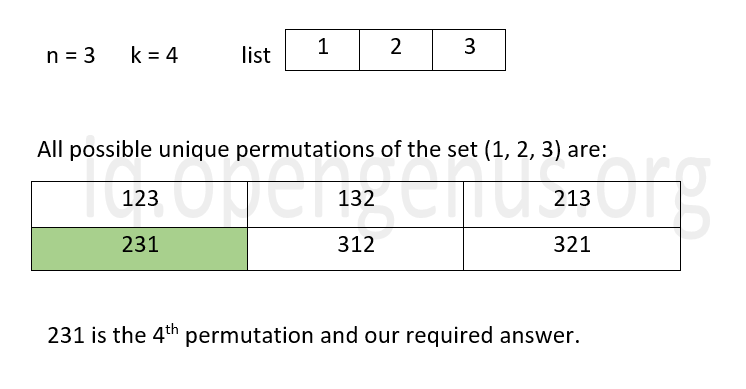There are two ways to tackle this problem.

One is to generate the permutation sequence for the set containing numbers from 1 to n, arrange them in the order that we want and then pick out the integer in the kth position directly for our answer. But this is a time consuming process. We need to generate the whole sequence to just find one number. Hence this method is not preferred and we opt for the second way to find the kth permutation.

The second method is to find a pattern in the permutation sequence that will eventually help us in finding the kth permutation without having to find all possible permutations. The algorithm to this method is discussed below.

### Algorithm

• Initialize the string result to store the answer.
• Initialze a list which contains values from 1 to n.
• While n>0:
* Find the number of permutations which is equal to (n-1)!
* Find the index at which the k exists as k/(n-1)!
* If k % (n-1)! =0, then index=index-1
* Add the value at index position in the list into result and remove the value from the list.
* Decrement n by 1
• Return the result.

## Working of the algorithm

Let us understand how this algorithm works with the help of an example.
Let n=4 and k=9 i.e we need to find the 9th permutation in the permutation sequence of set (1, 2, 3, 4).

• Initialization: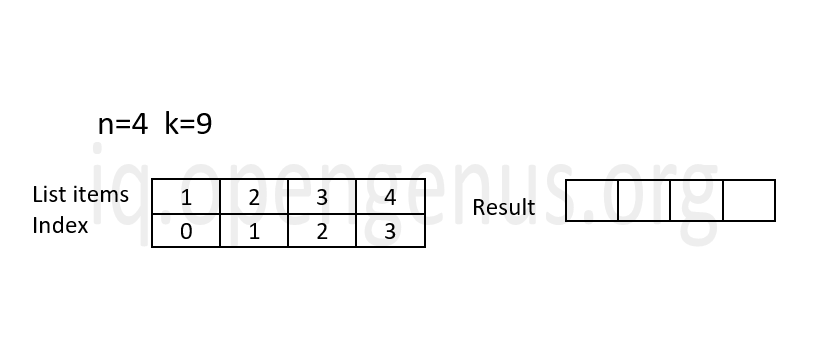• Iteration 1: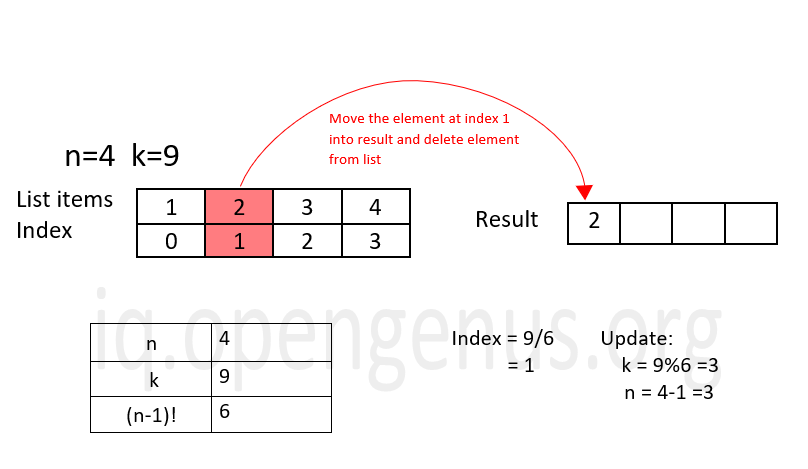• Iteration 2: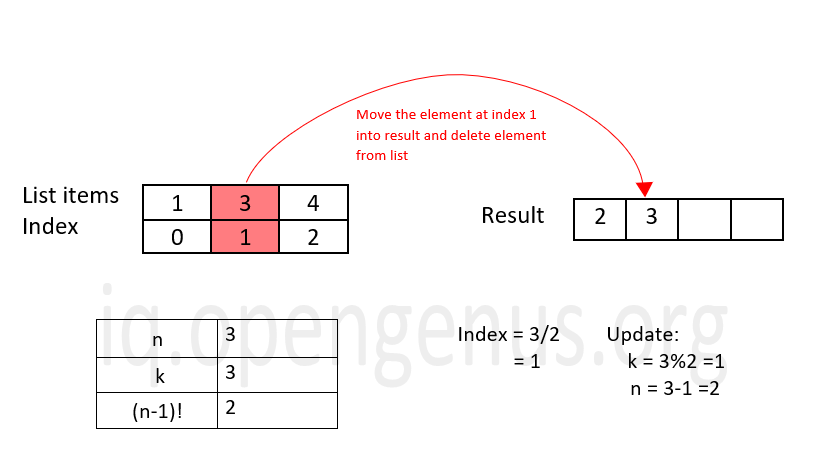• Iteration 3: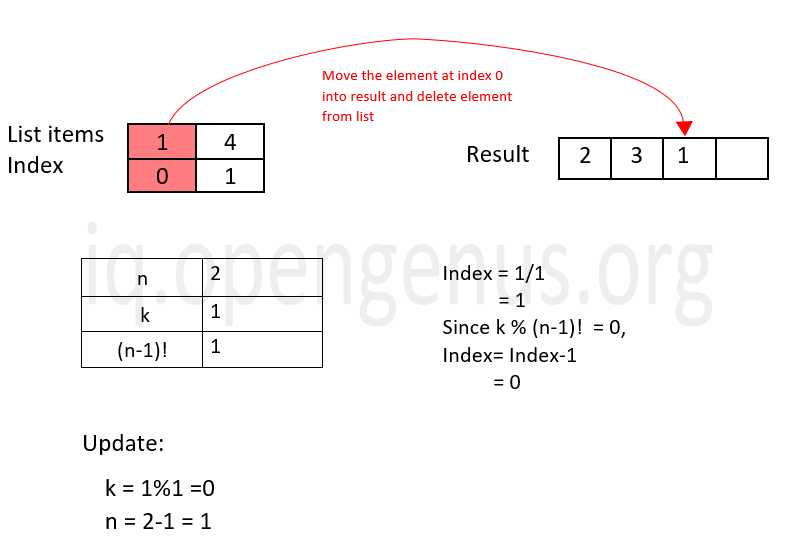• Iteration 4: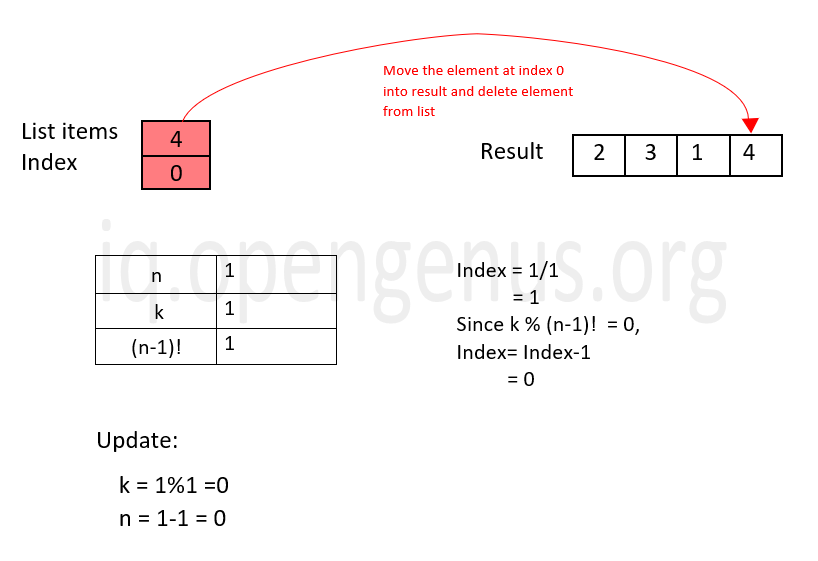Since, n=0 after iteration 4, the program comes out of the while loop and the result is printed. Therefore, the 9th permutation of set (1, 2, 3, 4) is 2314 as per the algorithm described.

## Python implementation

To implement the algorithm, we define two functions: findNumIndex() and findKthPermutation().

### Function to find the index of the element

``````def findNumIndex(k, n):
if (n == 1):
return 0, k
n -= 1    #so that we find factorial of (n-1)
num_index = 0
n_fact = n
#Calculating factorial of (n-1)
while (k >= n_fact and n > 1):
n_fact = n_fact * (n - 1)
n -= 1
#Calculating the index
num_index = k // n_fact
#Updating k value
k = k % n_partial_fact

return num_index, k

``````

We first define a function findNumIndex() that takes k and n as inputs. The factorial of (n-1) is then found by using a while loop with the condition n >1 and k is greater than or equal to the factorial. After each iteration of while loop, the value of n is decremented by 1. The function then calculates the index of the number, updates the value of k and returns both the index of the number and the k value.

### Function to find the kth Permutation

``````def findKthPermutation(n, k):

result = ""

s = set()

# Insert all natural number upto n in set
for i in range(1, n + 1):

# Subtracting 1 to get 0 based indexing
k = k - 1

for i in range(n):

array = list(s)

index, k = findNumIndex(k, n - i)

# array now points to the number at index in set s
result += str(array[index])

# remove current number from the set
array.pop(index)

s = set(array)

return result
``````

We then define a function findKthPermutation() that also takes n and k as inputs. An empty string result is created to store the final answer and an empty set s. Using for loop, all the numbers from 1 to n are inserted into the set. The k value is decremented by 1 to support the 0-based indexing followed in python.

Using for loop, we iterate over all the elements in set s and for each iteration, the following actions are executed:

• Convert the set s into a list and store it in a variable array.
• The index and k-value are found by calling the findNumIndex() function.
• The element in that index position in array is coverted to string and added into result.
• That particular element is deleted from array.
• array is converted back to a set.

The function then returns the result after it comes out of the for loop.

### Driver code

``````if __name__=='__main__':

n = 4
k = 9

kth_perm_seq = findKthPermutation(n, k)

print(kth_perm_seq)
``````

Here, we initialize the required n and k value and call the findKthPermutation() function to obtain the result.

### Output

``````2314
``````

# Complexity analysis

The algorithm discussed above in this article at OpenGenus has a time complexity of O(N) and space complexity of O(N) where N is the number of elements in the list array in the python implementation.#### Sanjana Babu

Sanjana Babu is a Student at SRM Valliammai Engineering College and is an Intern at OpenGenus. She is a Philomath.

K-th permutation of first N integers